Home > Blog > Artificial Intelligence > Top 80+ Machine Learning Interview Questions & Answers 2023

# Top 80+ Machine Learning Interview Questions & Answers 2023

27 Mar 2023
659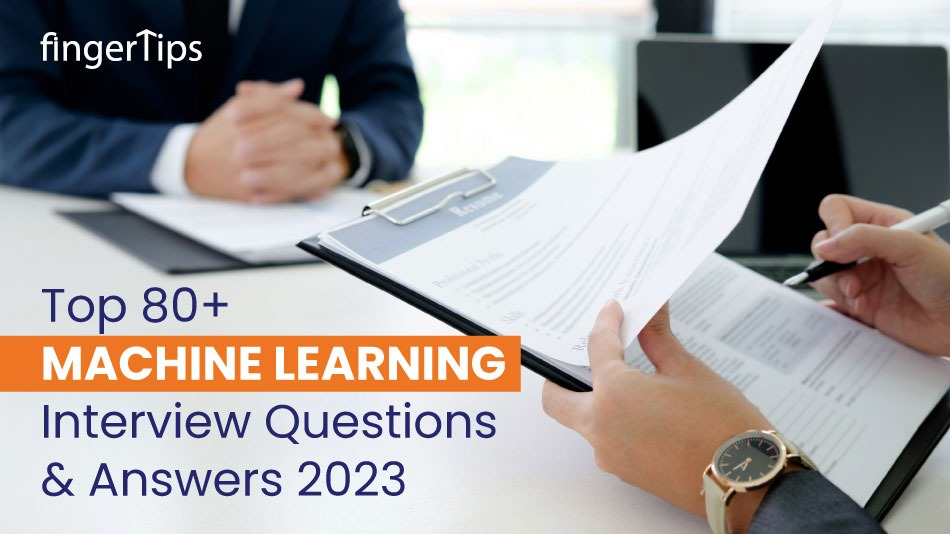### Related TopicsInterested in this course?Are you willing to bag a job in machine learning and make the best of the opportunity? This blog will guide you on how to face the machine learning interview questions. The series of questions in this blog will help you understand the concept and answer the interview questions on machine learning with confidence and ease. The machine learning interview questions prepared in this blog are based on the study and experience gained from many reputed companies that choose to ask particular machine learning interview questions.

Let’s begin!

Prepare to brush up on your skills and have a confident machine-learning interview experience. So, what exactly does machine learning refers to? It is a process of developing the algorithm that trains the computer program to create statistical database patterns. Machine learning aims to identify the fundamental patterns of data and turn the data to define essential data insights.

For example, if we have an authentic dataset of actual sales figures, we can program the machine learning models to forecast or prefigure future sales reports.

Why is the Machine Learning trend on the high rise?

Machine learning is the core and imperative part of artificial intelligence. With basic machine learning algorithms, you can solve practical real-time problems. All the necessary information is extracted from the data and it is used to solve the issue and predict future figures. In recent times the statistics state that 80% of enterprises practicing machine learning and artificial intelligence have significantly progressed in the business and experienced immense financial growth. Machine Learning solves Real-World problems. Machine learning algorithms learn from the data, unlike the hard coding rule to solve the problem.

Many reputed companies welcome talent from the machine learning field; the growth is high and will continue to be in demand. The interviewers seek talent with sound knowledge of machine learning algorithms that can help automate the task without explicit programming. The candidate`s competence is tested by checking on his areas of expertise. So, prepare to leave a positive impact on the interviewers by clearing all the machine learning interview questions with confidence.

This comprehensive blog highlights the top 80+ machine learning interview questions in 2023.

Basic Machine learning interview questions

The basic machine learning interview questions cover a wide range of machine learning questions that will prove very beneficial for the candidate to present the machine learning concept and basic machine learning algorithm.

1. What is Machine Learning?

This is one of the basic machine learning interview questions so specify that machine learning is a type of artificial intelligence that allows computer systems to learn from data, without being explicitly programmed. It involves building models that can recognize patterns in the data and make predictions or decisions based on those patterns.2. What are the stages of building a Machine Learning model?

The stages of building a machine learning model include:

•  Collecting and preprocessing the data
•  Splitting the data into training, validation, and test sets
•  Choosing an appropriate model and training it on the training data
• Evaluating the model on the validation data and tuning the model's hyper-parameters
•  Evaluating the final model on the test data

3. What is the difference between inductive and deductive learning?

Inductive learning involves learning from specific examples and making generalizations based on those examples. Deductive learning involves starting with general rules or principles and applying them to specific cases.

4. What is the difference between supervised, unsupervised, and reinforcement learning?

Keep a simple approach while answering this machine learning interview question, you can state that supervised learning involves training a model on labeled data, where the correct output is provided for each example in the training set. Unsupervised learning involves training a model on unlabeled data, where the model must find patterns in the data on its own.

Reinforcement learning involves training a model to make decisions in an interactive environment, where the model receives rewards or punishments based on its actions.

5. Out of model accuracy and performance which one is more important?

It depends on the context and the goals of the model. Accuracy is more important when the consequences of making a mistake are high, such as in medical diagnosis or financial prediction. Performance is more important when the model needs to make decisions in real-time, such as in a self-driving car or a recommendation system.

6. What is Bias- Variance tradeoff in Machine Learning?

The bias-variance tradeoff in machine learning refers to the tradeoff between the model’s ability to fit the training data well (low bias) and the model's ability to generalize to new data (low variance). A model with high bias will underfit the training data, while a model with high variance will overfit the training data.

7. Why do we need validation and test datasets?

Validation and test datasets are needed to evaluate the performance of a machine learning model. The validation set is used to tune the model’s hyper-parameters and evaluate its performance on unseen data. The test set is used to evaluate the final model's performance on completely unseen data.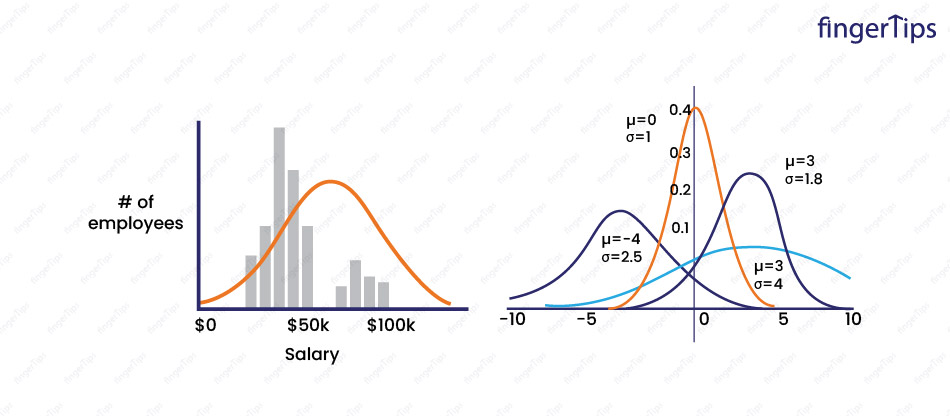8. What is the difference between Parametric and Non-parametric Models?

Parametric models make assumptions about the form of the underlying data distribution. Non-parametric models do not make assumptions and can be more flexible, but may also be more prone to over-fitting.

9. What are hyper-parameters, and how are they different from parameters?

Hyper-parameters are the parameters that control the behavior of a machine learning model, such as the learning rate or the number of hidden units in a neural network. They are set by the practitioner and are not learned from the data during training. Parameters are the values that are learned from the data during training.

10. What is Heteroscedasticity?

The variance of the error terms in a model that is not constant is known as heteroscedasticity. This can cause problems when estimating the model's parameters, as the assumptions of some estimation methods may not hold.

11. How can one determine which algorithm to be used for a given dataset?

There are several factors to consider when choosing an algorithm for a given dataset including the size and complexity of data, the type of task (classification, regression), and the desired level of interpretability. It is suggested to try several different algorithms and compare their performance on the data.

## Machine learning Interview Questions On EDA & FE

This section covers the machine learning interview questions on EDA & FE techniques along with the normalization and standardization in machine learning. All points explained in very simple methods for candidate to understand well.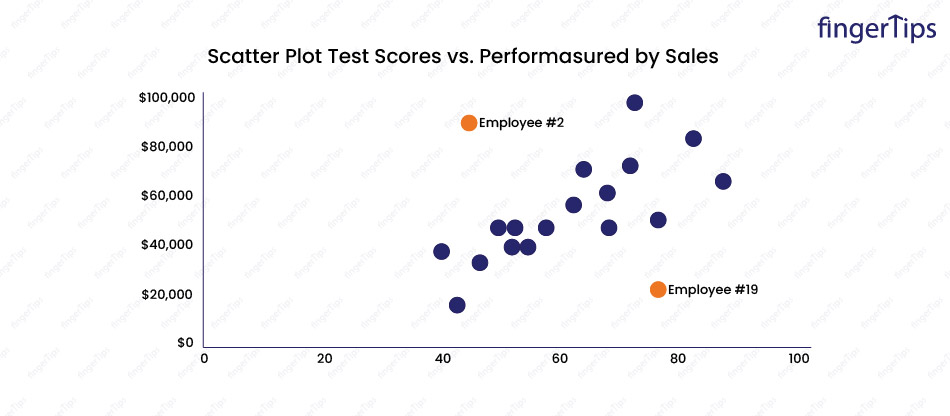12. What are some EDA techniques?

Exploratory Data Analysis techniques include visualizing data through plots and graphs, calculating summary statistics such as mean and standard deviation, and identifying patterns and trends in the data.

13. What is Cross-validation in Machine Learning?

Cross-validation is a method to evaluate the performance of a model by training it on a portion of the data and testing it on a different portion. This helps to prevent over-fitting, as the model is not just evaluated on the data it was trained on.

14. What are collinearity and multicollinearity?

Collinearity refers to the correlation between two or more predictor variables in a multiple regression model. Multicollinearity occurs when there is high collinearity among the predictor variables.

15. How to deal with multicollinearity?

There are several ways to deal with multicollinearity, including removing one or more of the highly correlated variables, using dimensionality reduction techniques such as principal component analysis (PCA), and adding regularization to the model.

16. What is the Variance Inflation Factor?

The Variance Inflation Factor is a measure of multicollinearity in a multiple regression model. It is calculated for each predictor variable and can be used to identify which variables may be causing multicollinearity.

17. What is PCA in Machine Learning?

Principal Component Analysis is a dimensionality reduction technique that projects the data onto a new set of orthogonal (uncorrelated) dimensions, known as principal components. We reduce number of dimensions and retain original variance as much as possible.

18. Why is rotation required in PCA? What will happen if the components are not rotated?

Rotation is required in PCA to ensure that the principal components are uncorrelated. If the components are not rotated, they may be correlated, which can affect the interpretation of the results.

19. What is meant by “Curse of Dimensionality”? List some ways to deal with it.

The "curse of dimensionality" refers to the challenges that arise when working with high-dimensional data, such as the need for a larger sample size and the difficulty in visualizing and interpreting the data. Some ways to deal with the curse of dimensionality include using dimensionality reduction techniques, such as PCA, and applying machine learning algorithms that are capable of handling high-dimensional data.

20. What is Dimensionality Reduction?

The process of reducing the number of dimensions (variables) in a dataset while preserving as much of the original information as possible is called Dimensionality reduction. This can be done for a variety of reasons, including improving model performance and reducing the complexity of the data.

21. What are some ways to Standardize Data?

Some ways to standardize data include scaling to have a mean of zero and a standard deviation of one and centering the data by subtracting the mean from each value.

22. What is the difference between Normalization and Standardization?

Normalization is the process of scaling the data to a specific range, such as [0, 1] or [-1, 1]. Standardization is the process of scaling the data to have a mean of zero and a standard deviation of one.

23. One-hot encoding increases the dimensionality of a dataset, but label encoding doesn’t. How?

One-hot encoding increases the dimensionality of a dataset by creating a new binary column for each unique category in a categorical variable. Label encoding does not increase the dimensionality of the dataset because it assigns a numerical value to each category in the variable, without creating new columns.

24. How can we handle an imbalanced dataset?

We can handle an imbalanced dataset by collecting more data, oversampling the minority class, under-sampling the majority class, and using algorithms that are specifically designed to handle imbalanced data.

25. What is a pipeline?

A pipeline is a series of steps that are performed on a dataset, like data preprocessing, feature selection, and model training. The goal is to automate the process and make it more efficient and reproducible.

## Machine Learning Interview Questions Regression

In this machine learning interview question section you will discover all about regression. Many of your concepts on regression will be explained in detail.

26. What is Linear Regression in Machine Learning?

Linear regression is a statistical method used to model the linear relationship between a dependent variable and one or more independent variables. Wetry to find a straight line (or a hyper-plane in the case of multiple independent variables) that best fits the data and can be used to make predictions about the dependent variable.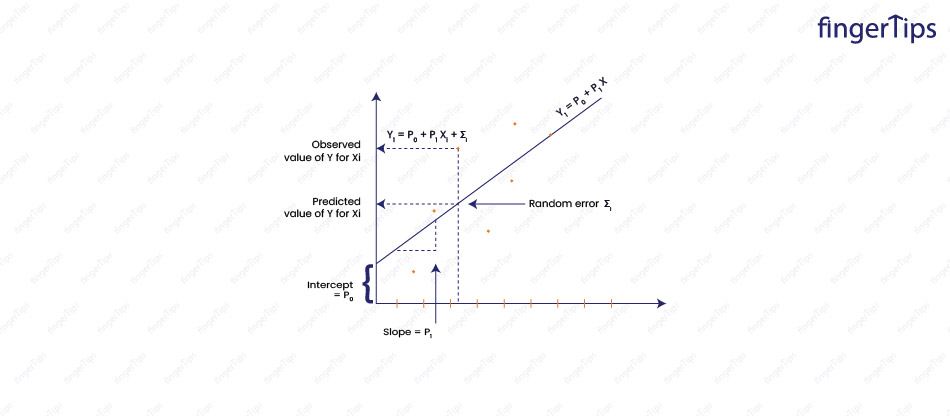27. What is the process of carrying out linear regression?

Be specific in explaining this machine learning interview question.

The process of carrying out linear regression involves the following steps:

• Collect and explore the data: This involves gathering the necessary data and performing exploratory data analysis.
• Prepare the data: This involves cleaning and preprocessing the data, such as handling missing values, scaling the variables, and splitting the data into training and test sets.
• Choose a model and train it: This involves selecting a suitable linear regression model (simple or multiple) and using the training data to estimate the model parameters.
• Evaluate the model: This involves using the test data to evaluate the performance of the model and assess its accuracy and predictive power.
• Fine-tune the model: The model can be fine-tuned by adjusting the parameters, adding or removing features, or using regularization.
• Make predictions: Once the model is trained and evaluated, it can be used to make predictions on new data.

28. Explain the difference between simple and multiple linear regression.

Simple linear regression involves only one independent variable, while multiple linear regression involves more than one.

Example: In simple linear regression, we try to predict the price of a house based on its size, while in multiple linear regression, we try to predict the price based on its size, location, number of rooms, etc.

29. Explain the concept of regularization and how it can be used in linear regression.

Regularization is a method to prevent over-fitting in linear regression by adding a penalty term to the objective function. The penalty term reduces the magnitude of the coefficients, which helps to reduce the complexity of the model and improve its performance.

There are two types of regularization used in linear regression:

• L2 or Ridge regularization: In this, we add a penalty term to the objective function that is proportional to the sum of the squares of the coefficients. The penalty term is controlled by a hyper-parameter called regularization strength or lambda. L2 regularization tends to produce models with small, non-zero coefficients, which can be useful for feature selection.
• L1 or Lasso regularization: In this, we add a penalty term to the objective function that is proportional to the sum of the absolute values of the coefficients. The penalty term is controlled by a hyper-parameter called regularization strength or alpha. L1 regularization tends to produce models with sparse coefficients, where many of the coefficients are exactly zero. This can be useful for feature selection and interpretation of the model.

Regularization is used to improve the performance of a linear regression model by reducing over-fitting. It is important to tune the regularization strength hyper-parameter to find the right balance between model complexity and error. Too much regularization can lead to under-fitting, while too little can lead to over-fitting.

30. What is the difference between Lasso and Ridge regression?

LASSO stands for Least Absolute Shrinkage and Selection Operator is a linear regression model that adds a penalty term to the objective function that is proportional to the sum of the absolute values of the coefficients:

Lasso objective function = MSE + alpha * ∑ |beta|

Where alpha is the regularization strength (a hyper-parameter) and beta is the coefficient for a feature.

Ridge regression adds a penalty term to the objective function that is proportional to the sum of the squares of the coefficients:

Ridge objective function = MSE + alpha * ∑ beta^2

31. When is ridge regression preferred over lasso?

Ridge regression and Lasso are both regularization techniques that can be used to prevent over-fitting in linear regression. Both methods add a penalty term to the objective function that reduces the magnitude of the coefficients, but they differ in the form of the penalty term.

Ridge regression is preferred when we want to include all the features in the model, but want to penalize large coefficients. This is useful when we have correlated features and want to include all of them in the model.

Lasso is preferred when we want to select a subset of the most important features and eliminate the less important ones. This is useful when we have a large number of features and want to reduce the complexity of the model.

It is suggested to try both Ridge and Lasso and compare the performance of the resulting models. The right regularization method will depend on the specific characteristics of the data and the goals of the analysis.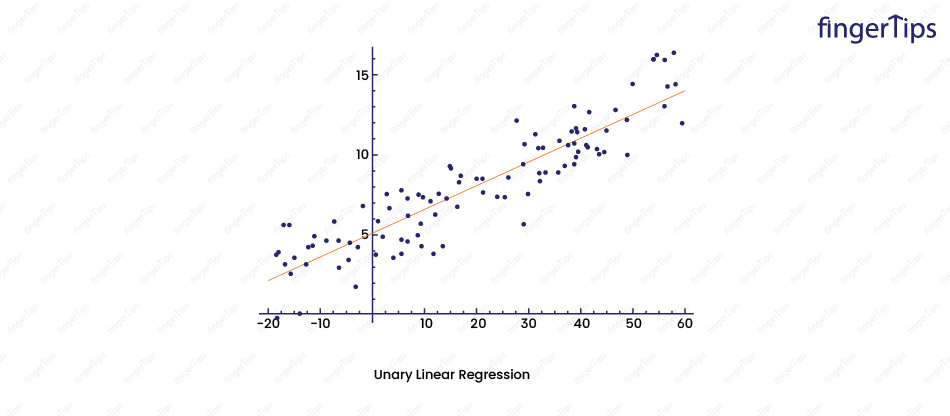32. Which performance metrics can be used to estimate the efficiency of a linear regression model?

There are several metrics used to estimate the efficiency of a linear regression model:

• R-squared: The variance in the dependent variable which can be explained by the model. More the value of R-square, better the fit is.
• Mean squared error (MSE): The average squared difference between the predicted values and the true values. A lower MSE value indicates a better fit.
•  Root mean squared error (RMSE): The square root of the MSE is more interpretable because it is in the same units as the dependent variable. RMSE value, better the fit is.
• Mean absolute error (MAE): This may be defined as the average absolute difference between predicted and true values. Lower the MAE value ,better the fit is.
• F-statistic: This statistic tests the hypothesis that the model is a significant improvement over a model with no independent variables. A higher F-statistic value indicates a better fit.
• Adjusted R-squared: An adjusted version of R-squared that takes into account the number of independent variables in the model. It is considered to be amore reliable metric than R-squared when we have a large number of independent variables.

No single metric is a perfect measure of model performance, and it's suggested to try multiple metrics to get a more complete picture of the model's efficiency.

33. Which performance metric is better R2 or adjusted R2?

R-squared and adjusted R-squared are both metrics that measure the proportion of the variance in the dependent variable that is explained by the model.

R2 = 1 - (SSE/SST)

SSE = sum of squared errors (i.e. the residual sum of squares)

SST= total sum of squares.

Adjusted R-squared is an adjusted version of R-squared that takes into account the number of independent variables in the model.

Adjusted R2 = 1 - (SSE/SST) * (n - 1)/(n - p - 1)

n = number of observations in the data set

p =the number of independent variables.

Both R-squared and adjusted R-squared are always between 0 and 1, and a higher value indicates a better fit.

Adjusted R-squared is generally considered to be a better metric because it is adjusted for the number of independent variables in the model and is therefore less prone to be overstated.

34. What is a Mean Squared error?

Mean squared error (MSE) is used to measure the quality of a linear regression model. It is the average squared difference between the predicted values (?) and the true values (y) for all the observations in the data set:

MSE = (1/n) ∑ (y - ?)^2

n = number of observations.

It is always non-negative, the smaller the value, the better the model. A model with an MSE of zero is a perfect fit, while a model with a large MSE has a large average error.

MSE is sensitive to the scale of the variables, so it is useful to scale the variables before calculating the MSE. It is also sensitive to outliers, so it is important to identify and handle outliers before calculating the MSE.

35. What is the error term composed of in regression?

In a regression model, the error term also known as the residual is the difference between the predicted value of the dependent variable and the true value.

error = y - ?

y= true value of the dependent variable

? = predicted value.

The error term is composed of two types of error:

• Irreducible error: Error that is inherent to the system being modeled and cannot be reduced by any means. For example, in the case of linear regression, the irreducible error is the error that is not explained by the independent variables.
• Reducible error: Error that is reduced by improving the model. It includes errors due to the limitations of the model (e.g. bias) and errors due to random noise in the data (e.g. variance).

36. How do you handle outliers in a linear regression model?

Outliers have a significant impact on the coefficients in a linear regression model, and sometimes even cause the model to be meaningless. One way to handle outliers is to simply remove them from the dataset before training the model. Another option is to use robust regression methods that are less sensitive to the presence of outliers.

37. How do you handle missing values in a linear regression model?

There are several approaches to handling missing values in a linear regression model:

• Deletion: Delete the rows with missing values
• Imputation: Impute the missing values using mean
• Prediction: Train a separate model to predict the missing values

## Machine Learning Interview Questions Classification

Explore all about classification in this machine-learning question list.

38. Explain Logistic Regression

Logistic regression is a supervised learning algorithm used for classification problems. It takes a set of input features and makes predictions about the likelihood of an event occurring. The predicted probability is transformed into a binary prediction using a threshold.

For example, if the predicted probability is greater than 0.5, the instance is classified as belonging to the positive class, and if it is less than 0.5, the instance is classified as belonging to the negative class.

The logistic function, which is used to predict the probability, is defined as follows:

p = 1 / (1 + exp(-z))

z = linear combination of the input features and the model weights

exp = exponential function.

Logistic regression is used for binary classification, where the goal is to predict one of two classes, or for multiclass classification, where the goal is to predict one of more than two classes.

39. Logistic regression is a classification technique and not a regression, why? Name the function it is derived from.

Logistic regression is a classification technique, not a regression as it is used to predict a class label and a continuous numeric output.

The name "logistic regression" can be misleading, as it is a classification algorithm and not a regression algorithm.

It is called "logistic" because it uses the logistic function (also known as the sigmoid function) to predict the probability that an instance belongs to a particular class.

The logistic function maps any real-valued number to a value between 0 and 1, which can be interpreted as a probability, and thus logistic regression is used for classification tasks.

40. Can logistic regression be used for classes of more than 2?

Yes, logistic regression can be used for multiclass classification tasks.

In the standard logistic regression, we predict the probability that an instance belongs to a particular class. The class with the highest predicted probability is then chosen as the predicted class. This is known as one-versus-all (OvA) classification or multiclass logistic regression.

For example, wehave a dataset with 3 classes: “good”, “better”, and  “best”. To perform multiclass logistic regression using the OvA method, we train three separate binary classifiers, one for each class:

• Classifier 1: good versus not good
• Classifier 2: better versus not better
• Classifier 3: best versus not best

To classify a new instance, we apply all three classifiers to the instance and choose the class with the highest predicted probability.

There are other approaches as well to multiclass logistic regression, such as one-versus-one (OvO) classification, which involves training a separate binary classifier for each pair of classes.

41. How would you evaluate a logistic regression model?

There are several ways to evaluate the performance of a logistic regression model:

• Accuracy: It is the proportion of correct predictions made by the model. It is calculated as the number of true positives and true negatives divided by the total number of instances. Accuracy can be misleading if the class distribution is imbalanced.
• Precision: It is the measure of the proportion of correct positive predictions. It is calculated as the number of true positives divided by the total number of positive predictions. Precision is useful for situations where false positives are more costly than false negatives.
• Recall: It is a measure of the proportion of actual positive instances that are correctly predicted by the model. Recall is number of true positives divided by the total number of actual positive instances. The recall is useful for situations where false negatives are more costly than false positives.
• F1 score: It is the harmonic mean of precision and recall, and is a good balance between the two. It is calculated as the harmonic mean of precision and recall, and is defined as F1 = 2 * (precision * recall) / (precision + recall)
• AUC-ROC: The AUC-ROC stands for the area under the receiver operating characteristic curve and is a metric that measures the ability of the model to distinguish between positive and negative classes. It is calculated by plotting the true positive rate against the false positive rate at various classification thresholds. A model with a high AUC-ROC score can correctly classify positive and negative instances more often than a model with a low AUC-ROC score.

42. Explain false negative, false positive, true negative, and true positive with an example.

• False negative: When a test incorrectly indicates that a condition is not present when it actually is present.
For example, if a medical test for a particular disease returns a negative result, but the patient actually has the disease, this is a false negative.
• False positive: When a test incorrectly indicates that a condition is present when it actually is not present.
For example, if a pregnancy test returns a positive result, but he is not actually pregnant, this is a false positive.
• True negative: When a test correctly indicates that a condition is not present.
For example, if a medical test for a particular disease returns a negative result, and the patient does not actually have the disease, this is a true negative.
• True positive: When a test correctly indicates that a condition is present.
For example, if a pregnancy test returns a positive result, and the person is actually pregnant, this is a true positive.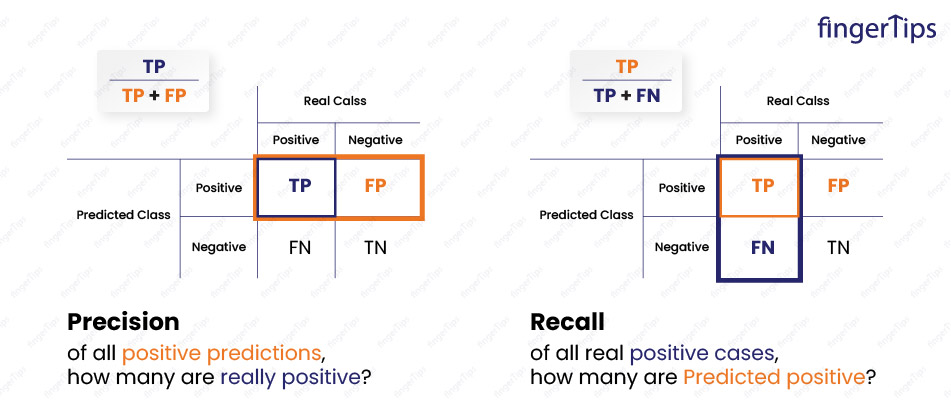43. What do you understand by Precision and Recall?

Precision and recall are two evaluation metrics to measure the accuracy of a classifier. For example, a binary classifier predicts whether an instance belongs to one of two classes (e.g., "positive" or "negative").

• Precision: This is the measure of the proportion of positive predictions that are actually correct. Number of true positives divided by the total number of positive predictions is called Precision. For example, if a classifier makes 100 positive predictions and 70 of them are correct, its precision is 70%.
• Recall: This is a measure of the proportion of actual positive instances that are correctly predicted by the classifier. Number of true positives divided by the total number of actual positive instances is known as Recall. For example, if there are 100 actual positive instances and the classifier correctly identifies 70 of them, its recall is 70%.

Both precision and recall are important in different situations.

For example, in a medical diagnosis setting, it is generally more important to have high recall (i.e., to minimize the number of false negatives, or missed diagnoses) even if it means accepting a higher number of false positives (incorrect diagnoses).

Whereas, in a spam filtering setting, it is generally more important to have high precision (i.e., to minimize the number of false positives, or non-spam emails that are classified as spam) even if it means accepting a higher number of false negatives (spam emails that are not detected).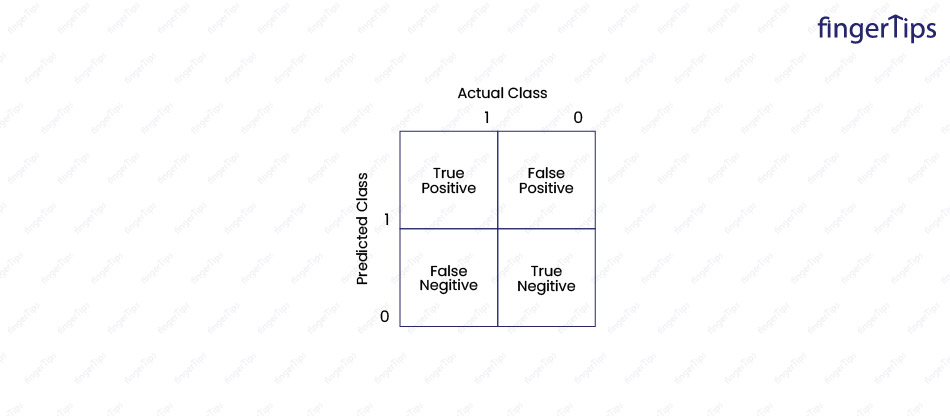44. What is a Confusion Matrix?

A table used to evaluate the performance of a classifier is known as Confusion Matrix. The rows of the confusion matrix correspond to the actual classes of the instances, and the columns correspond to the predicted classes. The confusion matrix contains counts or proportions of the instances in each category.

 Predicted Positive Predicted Negative Actual Positive True Positive (TP) False Negative (FN) Actual Negative False Positive (FP) True Negative (TN)

The counts in the confusion matrix can be used to compute various evaluation metrics, such as precision, recall, and accuracy.

45. What is the ROC curve and what does it represent?
A ROC curve (receiver operating characteristic curve) is a graphical plot that shows the performance of a binary classifier system as the classification threshold is varied. It is used for visualizing and comparing the performance of different classifiers, and for selecting a threshold that maximizes the desired performance metric.
The ROC curve plots the true positive rate (TPR) on the y-axis and the false positive rate (FPR) on the x-axis, for a range of possible classification thresholds.
A classifier that is performing well will have a curve that is close to the upper left corner of the plot, which indicates a high true positive rate and a low false positive rate. A classifier that is not performing well will have a curve that is closer to the diagonal line, which indicates a lower true positive rate and a higher false positive rate.

46. What is the Sigmoid function?
The sigmoid function maps areal-valued number to a value between 0 and 1. It is an activation function for a binary classifier, where the output values represent the probability of an instance belonging to each class.

47. What is F1-score and its use?
The F1 score is a metric to measure the performance of a classifier. It is a combination of precision and recall and is the harmonic mean of these two.
F1 = 2 * (precision * recall) / (precision + recall)
F1 score takes both precision and recall into account and is a good balance between the two.
F1 score is used in binary classification tasks, where we predict whether an instance belongs to one of two classes. It is also used in multiclass classification tasks, where the goal is to predict the class of an instance from a set of more than two classes.

48. What are the hyper-parameters of a logistic regression model?
The hyper-parameters of a logistic regression model are parameters that are set before training the model and controlling the learning process. Some common hyper-parameters are:
• Regularization: A technique used to prevent over-fitting by adding a penalty term to the objective function being optimized. The regularization term is a penalty on the L2 norm of the model weights, which is added to the negative log-likelihood loss. The strength of the regularization penalty is controlled by a hyper-parameter known as regularization strength, or lambda (λ).
• Learning rate: A hyper-parameter that controls the step size taken by the optimization algorithm during training. It determines how fast or slow the model learns from the training data. A smaller learning rate leads to a more accurate model, but it takes longer to train.
• Convergence tolerance: A hyper-parameter that determines the stopping criteria for the optimization algorithm. It is the maximum difference between the weights of two consecutive iterations that is allowed before the optimization is considered to have converged.
There are other hyper-parameters used to control the optimization process, such as the optimization algorithm (gradient descent, stochastic gradient descent) the batch size, and the number of epochs.

49. How do you deal with the class imbalance in a classification problem?
Class imbalance is a classification problem where the number of instances in one or more classes is significantly lower than the number of instances in the other classes. Because of this,a classifier trained on imbalanced data may be biased towards the more common classes, and may not perform well on the less common classes.
There are several techniques to deal with class imbalance:
• Collect more data: This can help the classifier learn more about the less common classes and may improve its performance.
• Resample the data: By either over-sampling the underrepresented classes or under-sampling the overrepresented classes. -
• Use balanced class weights: Classifier like logistic regression supports class weights, which is used to adjust the impact of each class on the optimization process. We set higher weights for the underrepresented classes that help the classifier give more emphasis during training.
• Use a different evaluation metric: We may use an evaluation metric that is less sensitive to class imbalance, like the F1 score.

## Machine Learning Interview Questions SVM

Know all about the SVM in this section of the machine learning interview questions. Machine learning algorithms are well explained with examples for better understanding.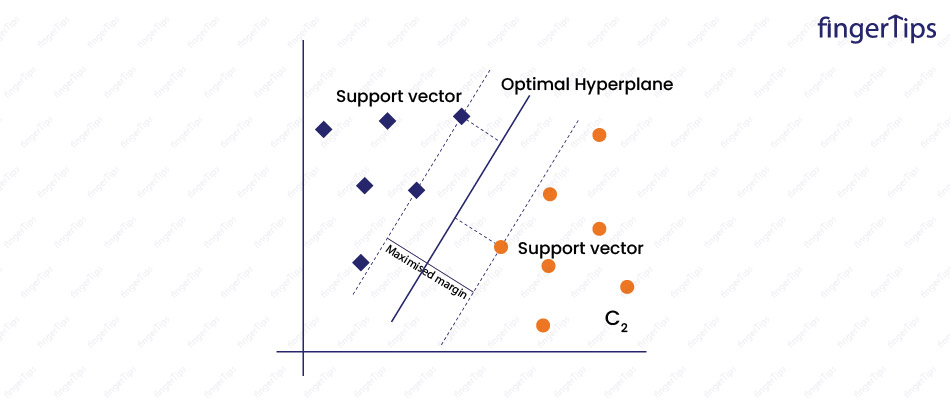50. What is Support Vector Machine?
It is a supervised machine learning algorithm used for classification or regression problems. SVMs are based on the idea of finding a hyper-plane that separates data points of different classes.
In classification problems, the SVM algorithm finds the hyper-plane that separates data points into different classes in a way that maximizes the margin between the hyper-plane and the data points. The distance between the hyper-plane and the nearest data points from each class is as large as possible. The hyper-plane is chosen in such a way that it has a maximum margin.
In regression problems, the SVM algorithm finds a hyper-plane that fits the data in a way that minimizes the error between predicted values and the true values.
SVMs are very effective in high-dimensional spaces and works fine with unstructured and semi-structured data. They are robust to over-fitting, which means they can generalize well to unseen data. However, they can be sensitive to the choice of kernel function and hyper-parameters and are computationally expensive to train large datasets.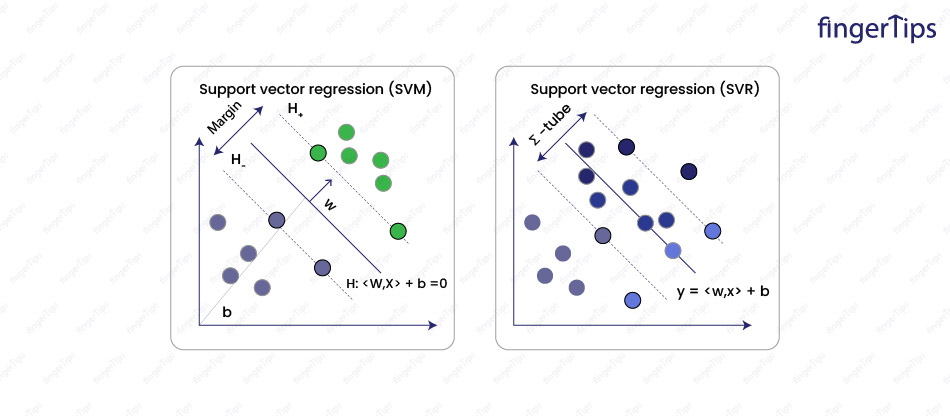51. What is the difference between SVC and SVR?
Support Vector Classification is a classification algorithm, while Support Vector Regression is a regression algorithm. Both are types of Support Vector Machines but are used for different types of problems.
SVC is used for classification problems, where we predict a categorical label for a given data point. For example, for a person (age, income, education level, etc.), the SVC algorithm is used to predict whether that person is likely to default on a loan.
SVR is used for regression problems, where we predict a continuous numerical value for a given data point. For example, on the size and number of rooms in a house, the SVR algorithm is used to predict the price of the house.

In both SVC and SVR, we find the hyper-plane that separates or fits the data points. The difference is loss function is used to measure the error between predicted values and true values. In SVC, the loss function is based on hinge loss, while in SVR, the loss function is based on epsilon-insensitive loss.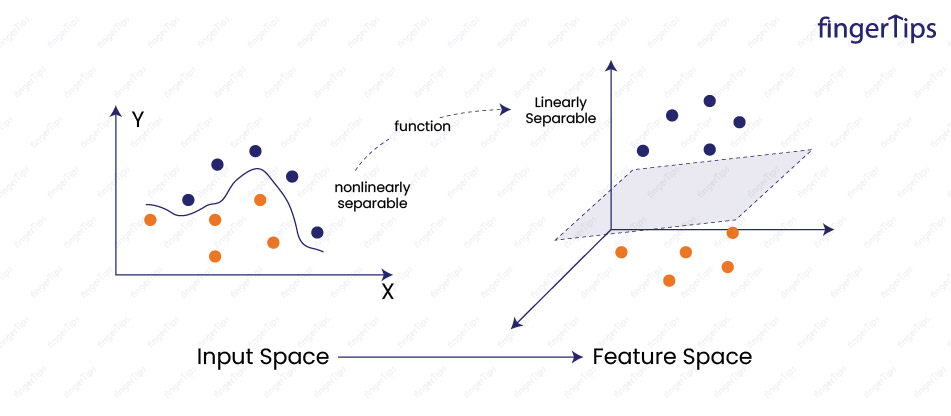52. What is Kernel SVM?
Kernel Support Vector Machine is a type of SVM that uses a kernel function to transform the data into higher-dimensional space, where it becomes possible to find a hyper-plane to separate the data points.
The kernel function creates additional features from the original features, which allows the SVM algorithm to capture more complex patterns in the data.
Kernel functions used with Kernel SVM are linear, polynomial, radial basis function (RBF), and sigmoid. The choice of kernel function impacts the performance of the model.

The advantage of Kernel SVM is that it can model non-linear relationships between the features and the target variable. This makes them a powerful tool for modeling complex data and is used in a variety of machine-learning tasks including image and text classification. However, they can be computationally expensive to train on large datasets.

53. What are the Various Kernels that are present in SVM?
There are various types of kernel functions used with Support Vector Machines:
• Linear: The simplest kernel function which calculates the dot product between input data points.
• Polynomial: A non-linear kernel function that captures relationships between variables that are not linear.
• Radial Basis Function (RBF): A non-linear kernel function that is defined as the exponential of the negative Euclidean distance between the input data points.
• Sigmoid: A non-linear kernel function.
The choice of kernel function can have a significant impact on the performance of the SVM model. It is a good idea to try out different kernel functions and evaluate their performance to find the one that works best for a given dataset.

54. What is the significance of Gamma and Regularization in SVM?
In Support Vector Machines, gamma is a hyper-parameter that determines the influence of a single training example on the decision boundary. A high value of gamma means that a single training example has a high influence on the decision boundary, and a low value of gamma means that a single training example has a low influence on the decision boundary.
In the radial basis function kernel, gamma is the coefficient that determines the shape of the decision boundary. A high value of gamma leads to a decision boundary that is more complex and flexible, and a low value of gamma leads to a decision boundary that is simpler and less flexible.
Regularization is a technique that is used to prevent over-fitting in machine learning models. It works by adding a penalty term to the objective function that is being optimized. The goal of regularization is to find a balance between the complexity of the model and the ability of the model to generalize to unseen data.

In the case of SVMs, the regularization term is an error term that is used to measure the difference between predicted and true values. A higher value of the regularization term means the model is less complex and more likely to generalize to unseen data, while a lower value means that the model is more complex and less likely to generalize.
The trade-off between complexity and generalization is controlled by the regularization parameter, which determines the strength of theregularization term. A higher value of the regularization parameter means the model is less complex and more likely to generalize, while a lower value means the model is more complex and less likely to generalize.

55. What is the difference between the normal soft margin SVM and SVM with a linear kernel?
A normal soft margin Support Vector Machine is used for classification problems and allows for a certain amount of misclassification of training data. The soft margin SVM finds a hyper-plane that separates the data points of different classes, while still allowing for some misclassification. This is done by introducing a slack variable that allows some data points to be on the wrong side of the hyper-plane.
The SVM with a linear kernel uses a linear kernel function. The linear kernel is the simplest kernel function, and simply calculates the dot product between the input data points.

The main difference is the type of kernel function that is used. The normal soft margin SVM can use any kernel function, while the SVM with a linear kernel is designed to use the linear kernel.
Another difference is that normal soft margin SVM allows for a certain amount of misclassification of the training data, while the SVM with a linear kernel does not. This means the normal soft margin SVM is more flexible and can model more complex patterns in the data, but may be more prone to over-fitting. The SVM with a linear kernel is less flexible but may be less prone to over-fitting.

56. What is the difference between soft and hard margins in SVM?
In Support Vector Machines, the margin is the distance between the decision boundary (the hyper-plane) and the nearest data points from each class.
A hard margin SVM tries to find a hyper-plane with the maximum possible margin and does not allow for any misclassification of training data.
A soft margin SVM allows for some misclassification of the training data. It finds a hyper-plane with a maximum margin but introduces a slack variable that allows some data points to be on the wrong side of the hyper-plane. The goal is to find a balance between a large margin and a low number of misclassified data points.

The main difference is the degree of tolerance for misclassification. A hard margin SVM does not allow for any misclassification, while a soft margin SVM allows for some misclassification.
Hard margin SVM is only possible when the data is linearly separable, which means it is possible to find a hyper-plane that perfectly separates the data points of different classes. However, it is rare to have data that is perfectly linearly separable so soft-margin SVM is usually used. Soft margin SVMs are more flexible and can model more complex patterns in data, but are more prone to over-fitting.
Image- hyper-parameters of SVM

57. What are the hyper-parameters of SVM?
There are several hyper-parameters in a Support Vector Machine model:
• Kernel: A hyper-parameter that is used to transform the data into a higher-dimensional space. Common kernel functions include linear, polynomial, radial basis functions, and sigmoid.
• Kernel coefficient: A hyper-parameter that is specific to the kernel function being used. For example, in the RBF kernel, the kernel coefficient is gamma, which determines the shape of the decision boundary.
• Regularization: A hyper-parameter that controls the complexity of the model. A higher value of the regularization means the model is less complex and more likely to generalize to unseen data, while a lower value means the model is more complex and less likely to generalize.
• Slack variables: In a soft margin SVM, these are hyper-parameters that control the degree of tolerance for misclassification. A higher value of the slack variables means the model is more tolerant of misclassified data points, while a lower value means the model is less tolerant.
Tuning these hyper-parameters have a significant impact on the performance of the SVM model, and it is necessary to try out different combinations to find the best values for a given dataset.

58. How can we handle imbalanced classes in an SVM model?
Imbalanced classes refer to classification problems where the number of data points in one class is much greater than the number of data points in another class. This isa problem for SVM models because they focus on data points that are closest to the decision boundary (support vectors), and these data points are from the majority class. As a result, the SVM model may be biased toward the majority class and may not perform well on the minority class.

There are several ways to handle imbalanced classes in an SVM model:
• Under-sampling: It involves reducing the number of data points in the majority class to be equal to the number of data points in the minority class. This can be done by randomly selecting a subset of the majority class data points.
• Oversampling: It involves increasing the number of data points in the minority class by sampling with replacement from the minority class data points.
• SMOTE: Synthetic Minority Over-sampling Technique (SMOTE) is an oversampling method that generates synthetic data points for minority classes by interpolating between existing minority class data points.
• Adjust class weights: By setting the class_weight parameter to a "balanced" model gives more importance to the minority class data points.
• Use a different loss function: For example,the "hinge" loss function is more sensitive to the misclassification of the minority class data points, while the "squared hinge" loss function is more sensitive to the misclassification of the majority class data points.

59. How do you evaluate the performance of an SVM model?
There are several ways to evaluate the performance of an SVM model:
• Accuracy
• Confusion matrix
• Precision
• Recall
• F1 score
• AUC-ROC curve
• Cross-validation

60. Can you discuss some of the limitations of using SVM for machine learning tasks?
There are several limitations to using SVM:
• It is sensitive to the selection of kernel and choice of hyper-parameters
• It is not well-suited for data sets with large numbers of features
• It is not robust to noise in data
• It is not easily interpretable
• It is not natively a probabilistic model

61. Can you give an example of a problem where an SVM would be a good choice for the algorithm?
Support Vector Machines (SVMs) are powerful and flexible machine-learning algorithms that can be used for a wide range of tasks. Some examples of problems where an SVM might be a good choice include:
• Anomaly detection
• Feature selection
• Non-linear boundary decision

62. What are the advantages of SVM algorithms?
There are several advantages to using Support Vector Machines:
• Effective in high-dimensional spaces
• Robust to over-fitting
• Versatile
• Efficient
SVMs is  powerful and versatile and used for a wide range of machine-learning tasks. However, they can be sensitive to the choice of kernel function and hyper-parameters and can be computationally expensive to train on large datasets.

## Machine Learning Interview Questions Naïve Bayes

Understand the Naive Bayes machine learning algorithm in this part of the machine learning interview question section.

63. What is Naive Bayes? Why is it called Naive?
Naive Bayes is a probabilistic algorithm that is based on the idea of using Bayes' theorem to make predictions. Inthe context of machine learning, Naive Bayes algorithms are used to make predictions based on the probability of certain events occurring given the presence of certain features in the data. For example, a Naive Bayes classifier might be used to predict the likelihood that a piece of email is spam based on the presence of certain words in the email.
Naive Bayes algorithms are called "naive" because they make a strong assumption about the independence of the features in the data.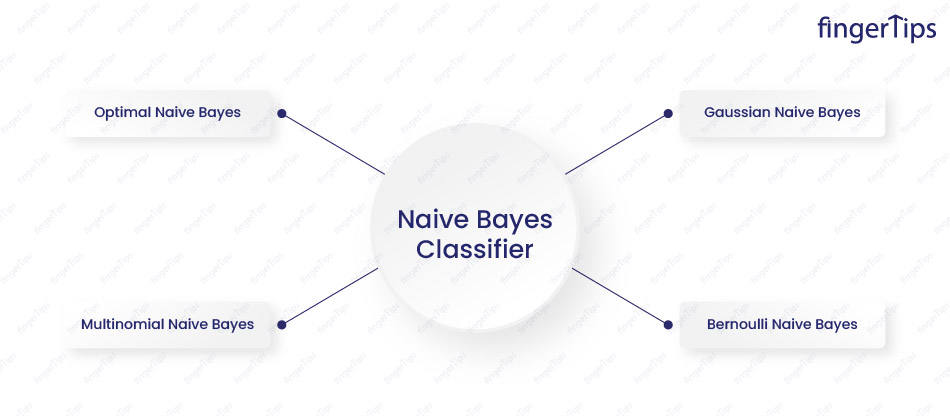64. Explain how a Naive Bayes Classifier works.
A Naive Bayes classifier is a probabilistic machine learning model used for classification problems. It predicts the probability of an instance belonging to each class and assigns the class with the highest probability.
Naive Bayes classifier works as follows:
• Calculates the prior probability of each class.
• Calculate the likelihood of each feature occurring given each class.
• Multiply the prior probability of each class by the likelihood of each feature to get the posterior probability of each class.
• The class with the highest posterior probability is the predicted class.
A Naive Bayes classifier assumes that features are independent of each other. The presence or absence of one feature does not affect the probability of any other feature.

65. Is naive Bayes a supervised or unsupervised machine learning algorithm?
Naive Bayes is a supervised machine learning algorithm that requires labeled training data to make predictions. During the training process, the algorithm estimates the probability of each class and the likelihood of each feature given to each class using the training data. It then uses these estimates to make predictions on new, unseen data.
In contrast, unsupervised machine learning algorithms do not require labeled training data. They try to discover patterns or relationships in the data without any prior knowledge of the output labels.

66. What are the advantages of using naive Bayes for classification?
There are several advantages to using a Naive Bayes classifier for classification:
• A simple and easy-to-implement algorithm.
• Fast and efficient, particularly for large datasets.
• Highly adaptive means it can adjust to changes in the distribution of the data.
• A probabilistic model means it outputs probabilities for each class label.
• Can achieve high accuracy even with a small amount of training data, and is often used as a baseline classifier.

67. Are Gaussian Naive Bayes the same as binomial Naive Bayes?
No, Gaussian Naive Bayes and binomial Naive Bayes are different.
Gaussian Naive Bayes is used for classification tasks where the features are continuous and follow a Gaussian (normal) distribution. It assumes that the likelihood of a feature given a class is Gaussian distribution.
Binomial Naive Bayes is used for classification tasks where the features are binary. It assumes that the likelihood of a feature given a class is a binomial distribution.

68. What is the difference between the Naive Bayes Classifier and the Bayes classifier?
The Naive Bayes classifier and the Bayes classifier are related but have distinct concepts.
The Bayes classifier is a theoretical classifier that makes predictions based on Bayes' theorem. It states that the probability of an event occurring is equal to the prior probability of the event multiplied by the likelihood of the event occurring given some evidence.
The Naive Bayes classifier isthe implementation of the Bayes classifier that makes a strong assumption about the independence of the features in the data. It assumes that the presence or absence of one feature does not affect the probability of any other feature.

69. What are the assumptions of naive Bayes?
The main assumption is the independence assumption, which states that the presence or absence of a particular feature is independent of the presence or absence of any other feature. This assumption is often unrealistic but allows the algorithm to make predictions with a relatively small amount of data and computational resources.

70. How can we evaluate the performance of a naive Bayes classifier?
The performance of a naive Bayes classifier can be evaluated using a variety of measures including accuracy, precision, and recall. These measures can be calculated using a test set of labeled data or using cross-validation techniques like k-fold cross-validation.

71. Describe a situation where naive Bayes might not perform well.
Naive Bayes might not perform well where the assumption of independence between features is significantly violated. It may also not perform well on datasets with alarge number of features or highly complex or non-linear relationships between features and labels.

72. Discuss any potential drawbacks or limitations of using naive Bayes.
Some drawbacks or limitations of naive Bayes include its reliance on the independence assumption, which may not hold in real and its simplicity which may not be sufficient to capture complex patterns in the data. It may also perform poorly on small or imbalanced datasets.

73. Give some real-world applications wherethe Naive Bayes classifier is used.
Naive Bayes classifiers are used in a wide range of real-world applications:
• Spam filtering
• Document classification
• Sentiment analysis
• Medical diagnosis
• Fraud detection
• Weather prediction

## Machine Learning Interview Questions KNN

In this part of the machine learning interview question and answer section, get to know in detail about the machine learning algorithm KNN and its specifications in detail.

74. What is the k-NN algorithm and how does it work?
k-NN is a supervised machine learning algorithm used for classification and regression. It identifies the k-number of training examples that are closest to the test example, and then classifies the test example based on the majority class among those k-training examples.

75. How to decide the value of k in k-NN?
The value of k in k-NN is chosen through cross-validation or using heuristics such as setting k to be the square root of the number of training examples.

76. How to handle missing or corrupted data in k-NN?
Missing data in k-NN is handled by imputing the missing values with the mean or median of the available values or by using techniques such as complete-case analysis or multiple imputations.

77. Which machine learning algorithm is known as the lazy learner, and why so?
The k-nearest neighbors (KNN) classifier is known as a "lazy learner" as it does not learn a discriminative function from the training data. It stores the training data and waits until it is asked to make a prediction. When a prediction is requested, the KNN classifier looks at k data points in the training set that is closest to the query point and returns the majority class among those k points as the prediction. Because the KNN classifier does not learn any internal representations from the training data, it is called a "lazy learner".

78. Is it possible to use KNN for image processing?
Yes, it is possible. We treat each pixel in an image as a data point and use the pixel values as features. You can then use a KNN classifier to predict the class of each pixel based on the values of its neighboring pixels.
For example, we can use a KNN classifier to classify each pixel in an image. To do this, we need to have a set of training images that have been labeled with the class of each pixel. We can then use these labeled training images to train a KNN classifier. Once the classifier is trained, we can use it to predict the class of each pixel in a new image.

79. Which distance do we measure in the case of KNN?
In the case of KNN, the distance between two data points is measured using a distance metric. There are many different distance metrics, and the choice of distance metric can affect the performance of the KNN classifier. Some distance metrics that are used with KNN include:
• Euclidean distance: The straight-line distance between two points. It is calculated as the square root of the sum of the squares of the differences between the coordinates of the points.
• Manhattan distance: This is also known as the "taxi cab" distance because it is the distance a taxi cab would need to travel to get from one point to another following a grid-like pattern. It is calculated as the sum of the absolute differences between the coordinates of the points.
• Cosine similarity: This is used when data points are represented as vectors. It measures the similarity between two vectors by calculating the cosine of the angle between them.
• Jaccard index: This is used when the data points are set. It measures the similarity between two sets by calculating the size of the intersection divided by the size of the union.
• Minkowski distance: This is a generalization of the Euclidean and Manhattan distances. It is calculated as the sum of the absolute differences between the coordinates of the points raised to the power of p, where p is a positive integer.
• Mahalanobis distance: This is used to account for correlations in the data. It is calculated using the inverse of the covariance matrix of the data.

80. How does the choice of normalization technique impact the model performance in k-NN?
Normalization scales the data such that all features are on the same scale. This improves the performance of the model. Common normalization techniques include min-max scaling and standardization.

81. How does the curse of dimensionality affect the performance of k-NN?
The curse of dimensionality refers to the fact that as the number of dimensions in the data increases, the number of data points needed to represent the data also increases exponentially. This can lead to poor performance of k-NN as the number of dimensions increases.

82. How to optimize the performance of k-NN?
The performance of k-NN can be optimized by choosing an appropriate value of k, and distance metric and normalizing the data.

83. Give an example of when k-NN might not be an appropriate algorithm to use.
k-NN is not an appropriate algorithm to use if the data has a large number of dimensions or if the data is noisy or has many outliers. It may also not be suitable for data with highly non-linear decision boundaries.

## Machine Learning Interview Questions Decision Trees

In this part of the machine learning interview question and answer section, explore the machine learning algorithm decision trees and its specifications.
Image- decision tree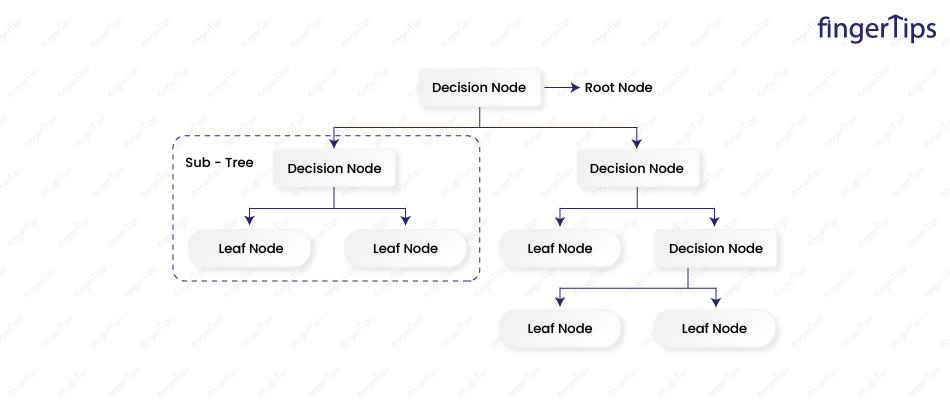84. What is a decision tree and how does it work?
It is a supervised ML algorithm used for classification or regression problems. It builds a tree-like model of decisions based on the features of the data. At each internal node of the tree, the model splits the data into two or more branches based on a decision rule and at each leaf node, a prediction is made based on the observations in that region.

85. How to determine which features to include in a decision tree?
We can use feature selection techniques such as selecting the features with the highest information gain or using a forward or backward selection approach.

86. How can we handle missing values in a decision tree?
We can replace the missing values with the mean or median value of the feature or we can use a decision tree algorithm that is capable of handling missing values, such as C4.5.

87. How can we prevent over-fitting in a decision tree?
One way to prevent over-fitting in a decision tree is to prune the tree by removing nodes that do not contribute to the prediction. Another way is to use a decision tree algorithm that includes regularization parameters, such as CART or C5.0.

88. What is the default method of splitting in decision trees?
The default method of splitting in decision trees is to use the Gini impurity index. The Gini impurity is a measure of how pure a node is.A pure node is one where all of the observations belong to the same class.

89. What is the difference between Gini Impurity and Entropy?
Gini impurity is a linear combination of the probabilities whereas entropy is a logarithmic function of the probabilities.
Entropy is more sensitive to changes in the probabilities and results in a more finely split tree.
Gini impurity is faster to compute and less prone to over-fitting but entropy may result in a more accurate model.

90. What is the difference between Entropy and Information Gain?
Entropy is a measure of disorder in a set of observations while information gain is a measure of a decrease in disorder that occurs when observations are split into subsets based on a feature.
Information gain is used to determine which feature to split on at each node in a decision tree, to select the feature that results in the largest decrease in entropy and the purest possible nodes.

91. Explain the process of pruning a decision tree.
Pruning a decision tree involves removing nodes from a tree to make the model simpler and more generalized to new data. For this, a decision tree is set to a minimum number of observations required at a leaf node or to remove nodes that do not improve the performance of the model on a hold-out validation set.

92. Why do we prune a tree?
There are several reasons to prune a decision tree:
• To prevent overfitting
• To improve interpretability
• To reduce computation time
• To improve prediction accuracy

93. Name some hyper-parameters of decision trees.
Some hyper-parameters for decision trees include:
• Maximum depth: Maximum number of nodes and the depth of the tree.
• Minimum samples per leaf: Minimum number of samples that must be present at a leaf node for the split to be considered.
• Minimum samples per split: Minimum number of samples that must be present at a node in for a split to be considered.
• Maximum features: Maximum number of features that can be considered when looking for the best split at each node.
• Maximum leaf nodes: Maximum number of leaf nodes that can be present in the tree. Smaller value results into a simpler tree.
• Criterion: Function that is used to evaluate the quality of a split.
• Splitter: The strategy that is used to select the split at each node.
• Class weight: Weight that is applied to each class in the training data.
• Presort: This determines whether or not to presort the data before building the tree.

Some advantages of decision trees are:
• Easy to understand and interpret
• Can handle high-dimensional data
• Can handle both continuous and categorical data
• Fast to train and make predictions

Some disadvantages of decision trees are:
• Can be prone to over-fitting
• May not be as accurate as other algorithms
• Can be sensitive to small changes in the data
• Can be unstablea. V

## Machine Learning Interview Questions Ensemble Learning/Forests

In this section of the machine learning interview question and answer section, you will find details on machine learning algorithm ensemble learning.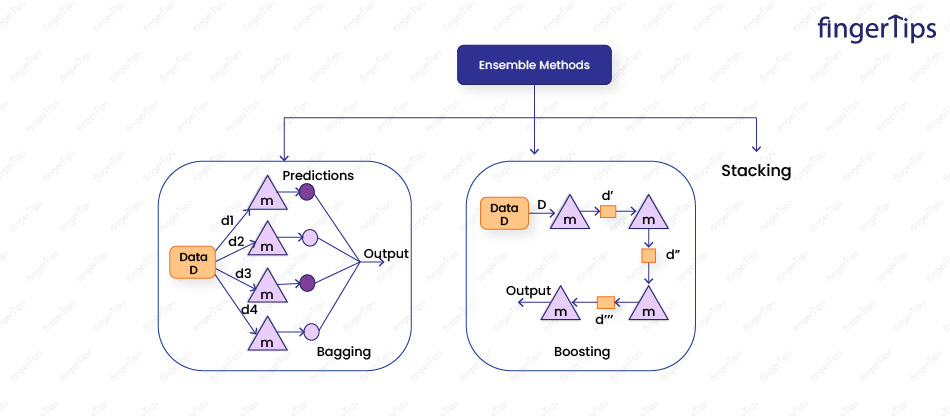95. Explain ensemble learning in Machine Learning.
Ensemble learning is a machine learning technique that combines the predictions of multiple models to make a more accurate final prediction. The goal is to improve the generalization performance of the model by training a diverse set of base models and then combining their predictions.
There are several ways to do this:
• Voting: Each base model makes a prediction, and the final prediction is made by taking the mode of the predictions for classification tasks orthe mean of the predictions for regression tasks.
• Averaging: Predictions of the base models are combined by taking the mean of the predictions in classification or regression tasks.
• Boosting: Base models are trained sequentially, correcting the mistakes made by the previous model. The final prediction is made by combining the predictions of all of the base models.
• Bagging: Multiple base models are trained on different subsets of input data. The final prediction is made by averaging the predictions made by the base models.
Ensemble learning is used with a variety of base models, such as decision trees, neural networks, and linear regression models. It is used to improve the accuracy of the model or to reduce the variance of the model to prevent over-fitting.

96. What is a random forest and how it is different from a single decision tree?
A random forest is an ensemble model that consists of multiple decision trees. It works by constructing a large number of decision trees and then aggregating the predictions made by each tree to make a final prediction.
A single decision tree is prone to over-fitting, and a random forest is less likely to overfit because the predictions made by the individual trees are averaged out.

97. How to choose the number of trees in a random forest?
The number of trees in a random forest is chosen through cross-validation or by using a heuristic such as the "out-of-bag" error, which estimates the error of the model using only data that was not used to train the individual trees.

98. How can we evaluate the performance of a random forest model?
The performance of a random forest model is evaluated using metrics such as accuracy, precision, and recall for classification problems or mean squared error and mean absolute error for regression problems. These metrics can be calculated using cross-validation or by using a separate test set.

99. What is the difference between bagging and boosting?
Bagging stands for bootstrap aggregating is an ensemble method in which multiple base models are trained on different subsets of the input data. The subsets of the data are created by sampling the original data with replacement, so each subset may contain duplicate data points. The base models are trained independently on the different subsets of the data, and the final prediction is made by averaging the predictions made by the base models. Bagging is a useful technique for reducing the variance of the model, which helps to prevent over-fitting.
Boosting is an ensemble method in which base models are trained sequentially, with each model attempting to correct the mistakes made by the previous model. The final prediction is made by combining the predictions of all of the base models. Boosting algorithms assign a higher weight to samples that were misclassified by the previous model, so that the next model in the sequence focuses more on these difficult samples. Boosting is a useful technique for improving the accuracy of the model, but it can be prone to over-fitting if the number of base models is not carefully controlled.

100. What is a voting model?
A voting model is a type of ensemble model in which multiple base models make predictions and the final prediction is made by taking the mode of the predictions for classification tasks or the mean of the predictions for regression tasks.
There are two main types of voting models:
• Hard voting: Each base model makes a prediction and the final prediction is made by taking the mode of the predictions.
• Soft voting: Each base model makes a probability prediction, and the final prediction is made by taking the mean of the probability predictions.
101. Which ensemble technique is used by Random forests?
Random forest uses bagging as the ensemble method.

102. Give a real-world application of random forests.
In the field of finance we can predict stock price movements or identify fraudulent credit card transactions, in the field of healthcare for predicting patient outcomes, and in the field of biology for predicting protein structures w can use random forests.

103. How to prevent over-fitting in a random forest?
Overfitting in a random forest can be prevented by limiting the depth of the individual trees or by pruning the trees to remove unnecessary branches or we can increase the number of trees in the forest, which will reduce the variance of the model.

104. What are the pros and cons of using a random forest versus a single decision tree?
The advantages of using a random forest over a single decision tree are that random forests are less prone to over-fitting, are more accurate, and can handle high-dimensional data.
The disadvantages of random forests are that they may be more computationally expensive to train and may not be as easy to interpret as a single decision tree.
Machine learning interview questions Algorithms Comparison
This particular section of the machine learning interview question and answer covers the machine learning algorithm comparison.

105. Differentiate between classification and regression in Machine Learning.
Classification is used to predict a discrete label or class for given input data.
Example: classification of spam mail
Regression is used to predict a continuous value for a given input data.
Example: predicting the price of a house given its features.

106. How is Random Forest different from Gradient Boosting, both being tree-based algorithms?
The difference between the two is in how the base models are trained.
In a random forest, the base models are trained independently on different random subsets of the training data, and the final prediction is made by averaging the predictions of all the base models.
In gradient boosting, the base models are trained sequentially, each model focusing on correcting the mistakes of the previous model. As a result, gradient boosting leads to more accurate predictions than random forests, but it can also be more computationally expensive and prone to over-fitting.

107. What is the difference between stochastic gradient descent and gradient descent?
Stochastic gradient descent(SGD) is a variant of gradient descent that uses a random subset of the training data, rather than the entire dataset to compute the gradient at each step.
This makes SGD faster and more scalable than gradient descent, but it can also be noisier and less stable. SGD is used when the training dataset is very large and cannot be fit in memory or when the cost function is very noisy.

108. Naive Bayes algorithm or decision trees, which one is better?
It depends on the specific problem and dataset. Naive Bayes is a simple and fast algorithm used for classification problems and works well when the features are independent of each other.
Decision tree scan handle more complex relationships between features and are used for both classification and regression problems.
Decision trees may be more powerful and flexible, but can also be more prone to over-fitting if not properly pruned.

109. Why does XGBoost perform better than SVM?
XGBoost is an implementation of gradient boosting that is designed for efficiency and scalability. It uses several techniques, such as sparsity-aware split finding, weight pruning, and block structure, to reduce the time and memory complexity of training a gradient-boosting model. Thus, it is often able to achieve higher performance than other gradient-boosting implementations, and also faster and more scalable.
Support vector machines (SVMs) are used for classification problems but are less efficient and less flexible than tree-based models such as XG-Boost.

## Scenario Based Machine Learning Interview Questions

Discover the scenario-based machine learning interview questions in this part of the blog.

110. Suppose you are working in an E-Commerce industry and your manager asked you to predict customers who will renew their subscription next month, what data will you need to do this? What analysis will you do and which algorithm will you use to build predictive models?
We need data on their past subscription behavior, also data on others like:
• Information about customers' subscription history like how long they have been a customer, how many subscriptions they have purchased in past, and whether they have consistently renewed their subscriptions in past.
• Demographic information such as age, gender, income level, and location.
• Usage data, including how often the customer uses the service and what features they use.
• Feedback or ratings provided by the customer as well as any interaction they had with customer support.
To analyze data, we want to get a sense of the overall pattern of subscription renewals and identify factors that seem to be important in predicting whether a customer will renew. Next, we build a predictive model that automatically predicts the customers who are likely to renew their subscriptions. We may choose algorithms like logistic regression, decision trees, and random forests. The choice of algorithm depends on the data and requirements of the problem.

111. You are working on a model which is suffering from low bias and high variance, which algorithm can fix this issue and why?
Bagging is an algorithm that can fix the issue of low bias and high variance in a model. It involves training multiple models on different subsets of data and then averaging the predictions of these models to obtain the final prediction. This can help reduce the variance of the model, at the cost of potentially increasing its bias.

112. You are working on a dataset having many variables some of which are highly correlated. You are asked to run PCA. Will you remove correlated variables first? If so, why?
It is a good idea to remove correlated variables before running PCA, as correlated variables can affect the results of PCA and make it difficult to interpret the principal components. Removing correlated variables can help reduce the dimensionality of the data, which can be useful if our goal is to reduce the number of variables in the model.

113. Suppose you want to build a multiple regression model but model R² is that good. You remove the intercept term and model R² becomes 0.75 from 0.3. Is it possible?
It is not possible to increase R²by removing the intercept term. The R-squared value measures the proportion of variance in the dependent variable that is explained by the model and removing the intercept term will not change the amount of variance explained by the model.

114. You build a random forest model with 10000 trees and got a training error of 0.00 but 34.23 on testing the validation. Have you trained your model perfectly?
No, a model with zero training error and high validation error is not well-trained. This is a sign of over-fitting, where the model has memorized the training data very well but is not generalizing to new data. It is important to have a good balance between training errors and validation errors to build a well-trained model.

115. What kind of recommendation system is used by ‘Amazon’ to recommend similar items to its customers?
Amazon uses a collaborative filtering recommendation system to recommend similar items to its customers. It is a method of making recommendations based on the past behavior of a group of users, rather than on the characteristics of the items themselves. The system looks at the items that users with similar tastes have purchased in the past and uses that information to make recommendations to other users.Fingertips